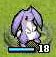# 星之一角

What have you found for these years?

## 2011-04-26

### powerset (4)

```powerset = filterM (const [True, False])
```

*

```powerset'' :: [a] -> [[a]]
powerset'' [] = [[]]
powerset'' (x:xs) = map (x:) (powerset'' xs) ++ (powerset'' xs)
```

```powerset''' :: [a] -> [[a]]
powerset''' [] = [[]]
powerset''' (x:xs) = do
flag <- [True, False]
ys <- powerset''' xs
if flag then
return (x:ys)
else
return ys
```

continuation 只有一個參數，就是原本的遞迴結果。

\r -> map (x:) r ++ r

tail recursion 的版本大概長這樣：
```powerset'''' :: [a] -> [[a]]
powerset'''' = (powerset''''rec (:[])) . reverse

powerset''''rec :: ([a] -> [[a]]) -> [a] -> [[a]]
powerset''''rec k [] = k []
powerset''''rec k (x:xs) = powerset''''rec (\r -> (map (x:) (k r)) ++ k r) xs
```

tail recursion > recursion = monad = concat map = filterM

filterM > tail recursion > monad > recursion = concat map

1. 改寫 tail recursion 是有意義的
2. 用最抽象的 filterM 去寫是有意義的...

stack overflow 的程式，改成這樣可能反而吃掉一堆記憶體，也讓 GC

#### 0 retries: